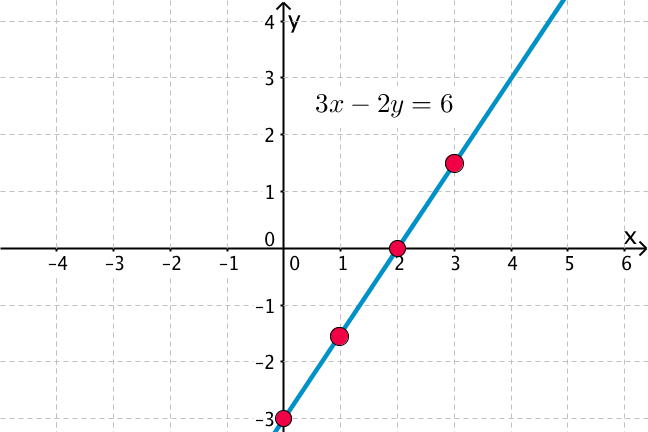## Want to keep learning?

This content is taken from the UNSW Sydney's online course, Maths for Humans: Linear and Quadratic Relations. Join the course to learn more.
1.9

## UNSW Sydney# Points and lines

Points and lines are abstract mathematical objects, but they have a direct physical interpretation on a Cartesian plane. While Euclid in his fundamental book The Elements was rather unclear about what a point and line were, with the rigid framework of Cartesian geometry we can pin down these ideas more precisely.

In this step we look at

• points and how to locate them

• lines and their linear equations.

## Points

For us the Cartesian plane is modelled by a sheet of graph paper, labelled with two axes, one horizontal called the $$\normalsize{x}$$-axis, and one vertical called the $$\normalsize{y}$$-axis. Each axis is marked by coordinates: usually only integer values (such as $$\normalsize{0,1,-1,2,-2}$$ etc) are shown, but we should be prepared to consider also fractional values. Although it is not necessary, the grid of equally spaced horizontal and vertical lines makes it easier to graph points and visualise functions.The official definition of a point is that it is an ordered pair of numbers enclosed in square brackets: such as the point $$\normalsize{A=[2,3]}$$. The first entry $$\normalsize{2}$$ is called the $$\normalsize{x}$$-coordinate of the point $$\normalsize{A}$$, while the second entry $$\normalsize{3}$$ is the $$\normalsize{y}$$-coordinate of $$\normalsize{A}$$. We agree to the convention that $$\normalsize{A}$$ is graphed as shown, moving from the origin horizontally to the right to the position $$\normalsize{x=2}$$ on the $$\normalsize{x}$$-axis, then vertically upwards to the position $$\normalsize{y=3}$$ on the $$\normalsize{y}$$-axis.

Q1 (E): which of the following points is not visualised on the graph below?

$$\normalsize{A=[2,3]}$$
$$\normalsize{B=[1,5]}$$
$$\normalsize{C=[-1,3]}$$
$$\normalsize{D=[-3,-2]}$$
$$\normalsize{E=[0,\frac{1}{2}]}$$
$$\normalsize{F=[0,4]}$$
$$\normalsize{G=[\frac{5}{2},-1]}$$Q2 (M): If you plotted that missing point, it would be exactly half way between which two points?

## Lines

The official definition of a line is that it is an equation of the form $$\normalsize{ax+by=c}$$, for example $$\normalsize 3x-2y=6$$. The coefficients are allowed to be any numbers (we will usually use integers, but fractions are fine too, and occasionally ‘irrational’ numbers such as $$\normalsize{\sqrt{2}}$$ turn up too). Just like points, lines have names, so we might write $$\normalsize{l:\; 3x-2y=6}$$.

We agree to the convention that the line $$\normalsize{l:\; 3x-2y=6}$$ is graphed by plotting those points $$\normalsize{[x,y]}$$ that actually satisfy the equation. Here are two such points: $$\normalsize{[2,0]}$$, $$\normalsize{[0,-3]}$$. They are arguably the simplest points to find, since plugging $$\normalsize{0}$$ into equations is generally pretty easy. In fact $$\normalsize{[2,0]}$$ is called the x-intercept of the line $$\normalsize{l}$$, while $$\normalsize{[0,-3]}$$ is called the y-intercept. For reasons that should be obvious to you, the $${\normalsize x}$$- and $${\normalsize y}$$-intercepts represent the points at which this line crosses the $${\normalsize x}$$ and $${\normalsize y}$$ axes.

Here are some additional points that satisfy our equation, arranged rather more systematically:

x y
3 1.5
2 0
1 -1.5
0 -3

Having all these points, we can plot the line $$\normalsize{l}$$ as shown:Since an equation can be manipulated algebraically, we will allow that the same line can be re-expressed in different forms.

Q3 (E): Which is the incorrect rewrite of $$\normalsize{l: 3x-2y=6}$$?

a) $$\normalsize{l:\; 6x-4y=12}$$

b) $$\normalsize{l:\; 3x-2y-6=0}$$

c) $$\normalsize{l:\; x=\frac{6+2y}{3}}$$

d) $$\normalsize{l: y=\frac{3}{2}x-3}$$

e) $$\normalsize{l: -4y-12=6x}$$

Now let’s have a look at some lines.Q4 (E): Which of the following lines does not appear in the above picture?

a) $$\normalsize{l:\; 3x-2y=6}$$

b) $$\normalsize{m:\; x+2y=3}$$

c) $$\normalsize{n:\; x=1}$$

d) $$\normalsize{o:\; y=x+1}$$

e) $$\normalsize{p:\; x=-y+2}$$

f) $$\normalsize{q:\; y=1}$$.

Q5 (M): If the missing line of the previous question were put in, it would go through the intersections of which lines?

## Relations between points and lines

Given a point such as $$\normalsize{A=[5,3]}$$ and a line $$\normalsize{l:\; 4x-3y=12}$$ there is a natural question to ask: does the point $$\normalsize{A}$$ lie on the line $$\normalsize{l}$$? Another way of saying this is to ask whether $$\normalsize{A}$$ is incident with $$\normalsize{l}$$. Another common equivalent phrase is that $$\normalsize{l}$$ passes through $$\normalsize{A}$$.

The beauty of this algebraic set-up is that the answer does not require any graphing of lines: it is purely an arithmetical issue of whether the two coordinates of $$\normalsize{A}$$ satisfy the equation of the line or not.

In this case if we substitute $$\normalsize{x=5}$$ and $$\normalsize{y=3}$$ into the line, we get $$\normalsize{20-9=12}$$ which of course is not a valid identity. Hence we conclude that $$\normalsize{A}$$ does not lie on the line $$\normalsize{l}$$.

A1. Only the point $$\normalsize{F=[0,4]}$$ is not shown.

A2. If you plotted $$\normalsize{F=[0,4]}$$ then it would be half way between $$\normalsize{B=[1,5]}$$ and $$\normalsize{C=[-1,3]}$$. Please note that you could see this algebraically too: the coordinate $$\normalsize{0}$$ is half-way between $$\normalsize{1}$$ and $$\normalsize{-1}$$, and the coordinate $$\normalsize{4}$$ is half way between $$\normalsize{5}$$ and $$\normalsize{3}$$. We say that $$\normalsize{F}$$ is the midpoint of the segment formed by $$\normalsize{B}$$ and $$\normalsize{C}$$.

A3. All are correct except e).

A4. The missing line from the picture is $$\normalsize{q:\; y=1}$$.

A5. If $$\normalsize{q:\; y=1}$$ were put in, it would go through the point $$\normalsize{[1,1]}$$, which also lies on the lines $$\normalsize{b,\;c,\;e}$$.